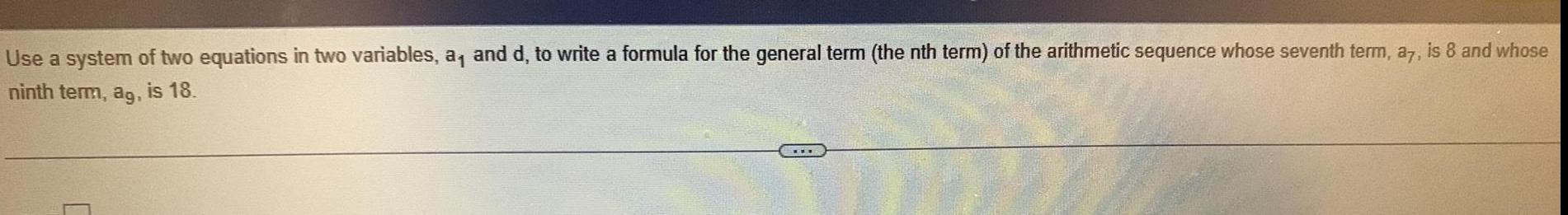Question:

# Use a system of two equations in two variables a and d to

Last updated: 11/16/2023Use a system of two equations in two variables a and d to write a formula for the general term the nth term of the arithmetic sequence whose seventh term a7 is 8 and whose ninth term ag is 18